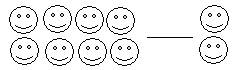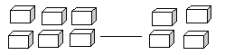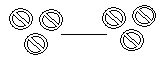Email us to get an instant 20% discount on highly effective K-12 Math & English kwizNET Programs!

#### Online Quiz (WorksheetABCD)

Questions Per Quiz = 2 4 6 8 10

### Grade 1 - Mathematics2.17 Greater Than and Less Than Using Pictures

 Explanation: The symbol for "Greater Than" is ">". The symbol for "Less Than" is "<". Method: Count the number of pictures in each set. If the first set has more pictures, write ">". If the first set has less pictures, write "<". If both sets have same number of pictures, write "=". Example: \$ \$ \$ \$ ___ % % % The number of \$'s is 4 and the number of %'s is 3. Since 4 is greater than 3, the answer is >. Directions: Answer the following questions. Also write at least 10 examples of your own.
 Q 1: Fill in the blank with greater than >, less than < or equal to =<=> Q 2: Fill in the blank with greater than >, less than < or equal to =>=< Q 3: Fill in the blank with greater than >, less than < or equal to =><= Q 4: Fill in the blank with greater than >, less than < or equal to =>=< Q 5: Fill in the blank with greater than >, less than < or equal to =<=> Q 6: Fill in the blank with greater than >, less than < or equal to =>=< Q 7: Fill in the blank with greater than >, less than < or equal to =>=< Q 8: Fill in the blank with greater than >, less than < or equal to ==>< Question 9: This question is available to subscribers only! Question 10: This question is available to subscribers only!